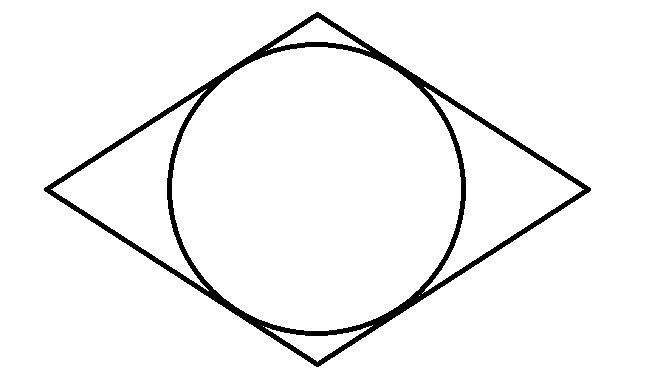A Circle Inside

Geometry Level 3A quadrilateral above has the same length of sides, has the same opposing angles and inscribes a circle whose circumference is $8\pi$. Determine the area of the quadrilateral whose smaller angles are equal to $60^{\circ}$.

Give your answer to 1 decimal place.

×